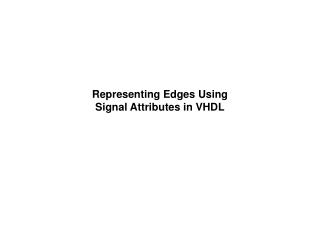# Representing Edges Using Signal Attributes in VHDL - PowerPoint PPT PresentationDownload PresentationRepresenting Edges Using Signal Attributes in VHDL

Representing Edges Using Signal Attributes in VHDL
Download Presentation## Representing Edges Using Signal Attributes in VHDL

- - - - - - - - - - - - - - - - - - - - - - - - - - - E N D - - - - - - - - - - - - - - - - - - - - - - - - - - -
##### Presentation Transcript

1. Representing Edges UsingSignal Attributesin VHDL

2. Attributes

3. Attributes (I/II)(pp. 75-76, Yalamanchili, "VHDL Starter's Guide") • signal_name'event returns true if there is a changein a value of the signal. • signal_name'active returns true if a value was assigned to the signal (even if the new value is the same as the current signal value). • signal_name'last_event returns the elapsed time since the last event that happened to the signal. • signal_name'last_active returns the elapsed time since the last time that the signal was active. • signal_name'last_value returns the last value that was assigned to the signal.

4. Attributes (II/II) • scalar_name'left returns the leftmost value in the definition of scalar_name’s data type. • scalar_name'right returns the rightmost possible value (in the data type) • scalar_name'high returns the highest possible value • scalar_name'low returns the lowest possible value • scalar_name'ascending returns true if the values in the definition are in increasing order. • array_name'length returns the number of elements in the vector

5. Attribute Examples in Detecting Clock Edges

6. Representing Edges inVHDL A clock change is represented using: CLK' event Which returns 'true‘ after the change to the clock. For a positive edge: CLK' event and CLK='1' Which returns ‘true’ after the change that led the clock to the value ‘1’ (from a value of ‘0’). For a negative edge: CLK' eventandCLK='0'

7. Edge Detection in the std_logic_1164 Package ------------------------------------------------------------------- -- edge detection ------------------------------------------------------------------- FUNCTION rising_edge (SIGNAL s : std_ulogic) RETURN BOOLEAN IS BEGIN RETURN (s'EVENT AND (To_X01(s) = '1') AND (To_X01(s'LAST_VALUE) = '0')); END; FUNCTION falling_edge (SIGNAL s : std_ulogic) RETURN BOOLEAN IS BEGIN RETURN (s'EVENT AND (To_X01(s) = '0') AND (To_X01(s'LAST_VALUE) = '1')); END; The functionTO_XO1(.) converts inputs to 'Χ', '0', or '1'. For detecting clock edges, we must use the functions given above!